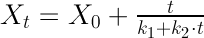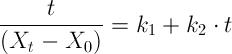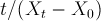### Goal: When you have finished this laboratory exercise you will understand

• how water absorbs in dehydrated foods during a rehydration process

### and you will learn

• how to determine water absorption in penne pasta at different temperatures, and
• to apply Peleg equation to experimental data in order to calculate the equation parameters and determine the equilibrium moisture content as a function of temperature.
• Removal of moisture from food products is an old and traditional preservation method.

Dehydrated foods are often rehydrated prior to their consumption or further processing in the industry.

During the rehydration process, as a dried product begins to absorb water, the process is affected by the medium temperature. Therefore in an industrial process, the water temperature during the rehydration process must be carefully controlled.

• Fresh Penne pasta purchased from a local market will be used in the experiments.

Prior to rehydration, pasta samples will be dried in an oven at 60oC for 1 hr, and dried samples will be soaked in the distilled water at different temperatures after recording their initial weight.

The rehydration experiments will be accomplished at 25, 50 and 75oC.

The soaked pasta samples will be removed from water at specified time intervals, blotted with a tissue paper and weighed to determine the amount of absorbed water.

The experiments will continue for 8 hr at each given temperature.

• Typical rehydration curves show an increase in the volume of the absorbed water, faster in the initial stages and slower in the latter stages. A typical rehydration curve is as follows:

• A commonly used mathematical model, known as Peleg's equation, contains a two parameter non-exponential equation:In this experiment, we will model the water absorption of Penne pasta by applying the Peleg's equation.

Data analysis will include mathematical approaches to determine the Peleg equation parameters and effect of temperature on the change of these parameters.

### Operator's Panel

2.28 kg/kg dry matter

### Virtual Experiment

• In this laboratory excercise, you obtained experimental data on the increase in the amount of absorbed water in Penne pasta with respect to immersion time. We will use the following procedure to determine the Peleg equation parameters:

1. The Peleg equation is given asIt can be re-written asshowing the linear trend ofversus immersion time.

• 2. After transferring the experimental data to an Excel spreadsheet, first the immersion time given in minutes will be converted to hours and the changes inwill be determined.

• 3. Then,will be plotted vs immersion time where the Trendline Option of Excel will be applied to get the slope and intercept of the trendline to determine Peleg equation parameters (k1 and k2).

• 4. Determination of the equilibrium moisture content: When a long immersion time is used, the Peleg equation becomes:

This resulting equation gives us the equilibrium moisture content (Xe) with the known initial moisture content (X0) and k2 values.

1. How did k1, k2 and Xe change with temperature? Did they follow any particular trends? Why?
2. How do you explain the increase of the equilibrium moisture content with water temperature? Was this an expected results? Why?
3. Review pertinent literature on the use of Weibull model for describing rehydration of foods and explain the mathematical procedure if the Weibull model is used to fit the experimental data.